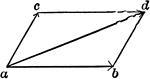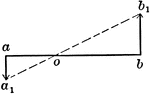### Motions Of 2 Points In Same Plane And Parallel

Illustration showing two points a and b to be in the same plane and parallel.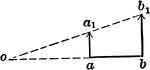### Motions Of 2 Points In Same Plane And Parallel

Illustration showing two points a and b to be in the same plane and parallel.### Motions Of 3 Points

Illustration showing three points a, b, and c in motion. The magnitude and direction of a and b are…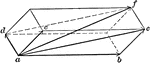### Parallelopiped of Motions

Illustration used "If three component motions ab, ac, and ad are combined, their resultant af will be…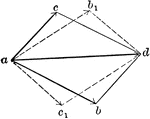Illustration "where ad is the given resultant, if the two components have the magnitudes represented…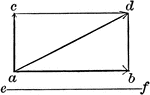### Vector Motion Into Two Components - Resultant

Illustration used to resolve a motion into two components, one of which is perpendicular, and the other…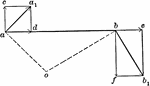### Velocities Of Rigidly-connected Points

Illustration for rigidly-connected points. "If two points are so connected that their distance apart…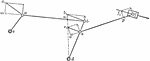### Velocities Of Rigidly-connected Points

Illustration for rigidly-connected points. In the series of links shown, c and d are fixed axes and…